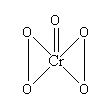QuestionAnswers

# In acidic medium, ${H_2}{O_2}$ changes $C{r_2}{O_7}^{2 - }$ to $Cr{O_5}$ which has two (-O-O-) bonds. Oxidation state of Cr in $Cr{O_5}$ is:(A) +5(B) +3(C) +6(D) -10Verified
128.7k+ views
Hint: In peroxide compounds, oxidation number of oxygen atoms is taken as -1 . We can calculate the overall charge of a compound by just adding respective oxidation numbers of all atoms present in the compound. From this expression, we can find the oxidation number of Cr.

Let’s see the structure of $Cr{O_5}$ to get ahead.You need to remember that $Cr{O_5}$ contains two -O-O- linkages. So, in short $Cr{O_5}$ has four oxygen atoms that are in peroxide linkage.
Now that is a rule that if oxygen atom is involved in a peroxide linkage, then both the oxygen have oxidation number +1 which is different from the normal oxidation state of oxygen atom.
Let’s calculate the oxidation state of Cr in $Cr{O_5}$.
Overall charge on compound= Oxidation state of Cr + 4(Oxidation state of oxygen in peroxide form) + Oxidation state of other oxygen atom
0 = Oxidation state of Cr + 4(-1) + (-2)
0 = Oxidation state of Cr - 4 - 2
Oxidation state of Cr = 4 + 2
Oxidation state of Cr = +6
Hence we can say that the correct answer of this question is (C) +6.